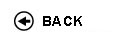Politecnico di Torino
Academic Year 2014/15
01NNEOV, 01NNENG
Discrete event models and systems
Master of science-level of the Bologna process in Computer Engineering - Torino
Master of science-level of the Bologna process in Mathematical Engineering - Torino
 Teacher Status SSD Les Ex Lab Tut Years teaching Calafiore Giuseppe CarloO2 ING-INF/04 30 30 0 0 5
 SSD CFU Activities Area context ING-INF/04 6 C - Affini o integrative A11
Esclusioni:
01NPQ; 01NPR; 04CFI; 01PDC; 01PDX; 01OUV
 Subject fundamentals The course is taught in Italian. Dynamical processes arising in various contexts, such as robotics, factory automation, networks, and economical systems, do not only possess a "continuous" behavior (i.e., the one which is typically studied in classical courses on systems and control theory), but also contain a "discrete" behavior, produced by the occurrence of asynchronous "events" (for instance, a component’s failure) that may modify instantaneously the system’s state. The analysis of systems of discrete nature requires tools and models that are quite different from the ones used in the traditional study of systems with continuous states. The purpose of this course is to introduce the basic elements necessary to understand the modeling of discrete event systems, to develop the relative theory, both in a deterministic and in a stochastic setting, and to analyze quantitatively their behavior, via an analytic approach or via a computer simulation one. Expected learning outcomes Understanding of analytical instruments for representing discrete event dynamical systems, both in a deterministic and a stochastic setting; Learning to model simple practical problems arising from factory automation, robotics, production systems and management; Acquiring the capability of evaluating a system’s performance (analytically or via computer simulation) and of dimensioning the system’s parameters, in the design phase; Understanding the behavior of networked systems. Prerequisites / Assumed knowledge Basic knowledge of calculus, probability theory, and linear algebra. Some exposure of systems and control theory may be useful, although it is not strictly required as a prerequisite. Contents - Discrete event dynamical systems (DEDS) modeling: states, events, transitions, graphs. - Review of probability theory and linear algebra. - DEDS, deterministic and stochastic timed automata, formalisms. - Computer simulation of DEDS. - Stochastic processes; Poisson, Exponential, and Gamma distributions. - Discrete-time and continuous-time Markov chains. - Queueing systems. - Open and closed networks of queues. Solution methods. - Network flow problems. - Examples from applicative contexts. Delivery modes The course is organized into lectures and practice sessions. Practice sessions are based on "exercise sheets" in which students are required to solve either analytical problems, or problems that require the use of a computer in order to simulate, estimate, evaluate and optimize a system’s characteristics (using StateTra software for DEDS modeling and simulation, and Matlab for general data processing). Texts, readings, handouts and other learning resources The course is based on the textbook indicated below, integrated by handouts that will be made available online by the instructor. Textbook: G. Calafiore, "Elementi di Automatica," CLUT (II edizione). Assessment and grading criteria Credits for this course are acquired upon successful completion of a written examination. The final grade will be based on the score obtained in the written exam, possibly weighted by an evaluation of the activity performed during the practice sessions. Programma definitivo per l'A.A.2014/15© Politecnico di Torino
Corso Duca degli Abruzzi, 24 - 10129 Torino, ITALY